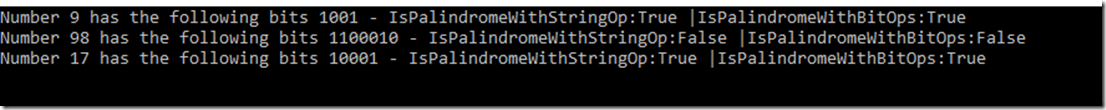# Binary Palindrome check in C#

Hi Community,

This post is about how to check whether the binary representation of a byte is a palindrome. I’ve recently received an email by a fellow developer requesting some help with this, and after having done a bit of research I couldn’t find any good example written in C#, so I decided to do it and share it with you. Similar to any other programming task and faithful to the phrase “There’s more than a way to skin a cat”, there are at least a couple of ways to do this via:

For the sake of clarity and completeness, I have written a code snippet to demonstrate both approaches, and they were implemented as extension methods for byte type as shown below

`public static class Binary {`

`    /// <summary>`

`    /// Gets the bits.`

`    /// </summary>`

`    /// <param name="a">a.</param>`

`    /// <returns></returns>`

`    public static string GetBits(this byte a) {`

`        var retval = string.Empty;`

` `

`        if (a > 0) {`

`            var buffer = new StringBuilder();`

`            var bitArray = new BitArray(new[] { a });`

` `

`            for (var index = bitArray.Count - 1; index >= 0; index--)`

`                buffer.Append(bitArray[index] ? "1" : "0");`

` `

`            retval = buffer.ToString().Substring(buffer.ToString().IndexOf("1", StringComparison.OrdinalIgnoreCase));`

` `

`        }`

` `

`        return retval;`

`    }`

` `

` `

`    /// <summary>`

`    /// Determines whether [is palindrome with string op].`

`    /// </summary>`

`    /// <param name="a">a.</param>`

`    /// <returns></returns>`

`    public static bool IsPalindromeWithStringOp(this byte a) {`

`        var retval = false;`

`        var bitStr = GetBits(a);`

` `

`        if (!string.IsNullOrEmpty(bitStr)) {`

`            var reversed = new string(bitStr.Reverse().ToArray());`

`            retval = reversed == bitStr;`

`        }`

` `

`        return retval;`

`    }`

` `

`    /// <summary>`

`    /// Determines whether [is palindrome with bit ops].`

`    /// </summary>`

`    /// <param name="a">a.</param>`

`    /// <returns></returns>`

`    public static bool IsPalindromeWithBitOps(this byte a) {`

`        var retval = false;`

` `

`        if (a > 0) {`

`            var leftShift = 0;`

`            var tempValue = (int)a;`

` `

`            while (tempValue != 0) {`

`                leftShift = leftShift << 1;`

`                var bitCheck = tempValue & 1;`

`                tempValue = tempValue >> 1;`

`                leftShift = leftShift | bitCheck;`

`            }`

`            retval = (leftShift ^ a) == 0;`

`        }`

` `

`        return retval;`

`    }`

Both methods are invoked

`var values = new byte[] { 9, 98, 17 };`

` `

`Array.ForEach(values, b => Console.WriteLine(\$"Number {b} has the following bits {b.GetBits()} - IsPalindromeWithStringOp:{b.IsPalindromeWithStringOp()} |IsPalindromeWithBitOps:{b.IsPalindromeWithBitOps()} "));`

` `

`Console.ReadLine();`

to check they return the same expected resultRegards,

Angel